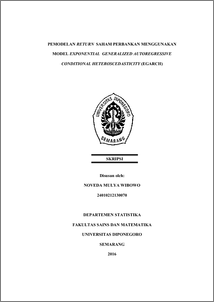# PEMODELAN RETURN SAHAM PERBANKAN MENGGUNAKAN MODEL EXPONENTIAL GENERALIZED AUTOREGRESSIVE CONDITIONAL HETEROSCEDASTICITY (EGARCH)

WIBOWO, NOVEDA MULYA (2017) PEMODELAN RETURN SAHAM PERBANKAN MENGGUNAKAN MODEL EXPONENTIAL GENERALIZED AUTOREGRESSIVE CONDITIONAL HETEROSCEDASTICITY (EGARCH). Undergraduate thesis, Fakultas Sains dan Matematika, Undip.Preview
PDF
1092Kb

## Abstract

ARIMA model is basically one of the models that can be applied in the time series data. In this ARIMA model, there is an assumption that the error variance of this model is constant. The price of stocks of the time series financial data, especially return has the trend to change quickly from time to time and it is actually fluctuative, so its error variance is inconstant or in another word, it calls as heteroscedasticity. To overcome this problem, it can be used the model of Autoregressive Conditional Heteroscedasticity (ARCH) or Generalized Autoregressive Conditional Heteroscedasticiy (GARCH). Furthermore, the financial data commonly has the different effect between the value of positive error and negative error toward the volatility data that is known as asymmetric effect. Indeed, one of the models used in this research, to overcome the problem of either heteroscedasticity or asymmetric effect toward the return of the close-stocks price of Banking daily is GARCH of asymmetric model that is Exponential Generalized Autoregressive Conditional Heteroscedasticity (EGARCH). The data of this research is the return data of the close-stocks price of Banking in October 31st 2013 to August 24th 2016. From the result of this analysis, it is gained several models of EGARCH. ARIMA model ([2,4],0,[2,4])-EGARCH (1,1) is such a best model for it has the lowest AIC value than any other models. Keywords: Return, Heteroscedasticity, Asymmetric effect, ARCH/GARCH, EGARCH

Item Type: Thesis (Undergraduate) H Social Sciences > HA Statistics Faculty of Science and Mathematics > Department of Statistics 55065 Mr Hasbi Yasin 26 Jul 2017 09:17 26 Jul 2017 09:17

Repository Staff Only: item control page